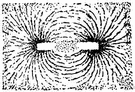# magnetic dipole moment

Also found in: Thesaurus, Medical, Encyclopedia, Wikipedia.

## magnetic dipole moment

n
(General Physics) a measure of the magnetic strength of a magnet or current-carrying coil, expressed as the torque per unit magnetic-flux density produced when the magnet or coil is set with its axis perpendicular to the magnetic field. Symbol: m or j Also called: magnetic moment Compare electromagnetic moment
Collins English Dictionary – Complete and Unabridged, 12th Edition 2014 © HarperCollins Publishers 1991, 1994, 1998, 2000, 2003, 2006, 2007, 2009, 2011, 2014
ThesaurusAntonymsRelated WordsSynonymsLegend:
 Noun 1magnetic dipole moment - (physics) a current loop gives rise to a magnetic field characteristic of a magnetic dipole; "An orbiting electron in an atom will have a magnetic dipole moment"natural philosophy, physics - the science of matter and energy and their interactions; "his favorite subject was physics"dipole moment - the moment of a dipole
Based on WordNet 3.0, Farlex clipart collection. © 2003-2012 Princeton University, Farlex Inc.
References in periodicals archive ?
The nuclear spin of the hydrogen proton of human body produces the magnetic dipole moment. In MRI, a strong DC magnetic field is applied to make the magnetic dipole moments process around the direction of the DC magnetic field with the procession frequency proportional to the magnetic field strength.
The detumbling module of the algorithm is based on the popular B-dot control, here rearranged to explicitly generate a magnetic dipole moment constantly orthogonal to the geomagnetic field vector.
(Electrons don't spin, it is just shorthand for "maintaining their magnetic dipole moment.")
In the SM, the prediction for the magnetic dipole moment (MDM) of the top quark is [a.sup.SM.sub.t] = 0.02 , which can be tested in current and future colliders, such as LHC and CLIC.
For the given magnetic dipole moment [M.sub.2], power transfer occurs through the time-varying magnetic field component in the direction of the moment.
Also here we analyze similar dependencies for the magnetic dipole moment. The influence of the electric field on the AB oscillation and the nanotubes magnetic dipole moment is considered in Section 4.
In the (7), r represents the inter-well distance; z represents vertical offset; R can be calculated by R = [square root of ([r.sup.2] + [z.sup.2])]; M is the magnetic dipole moment; [omega] is the angle frequency and [mu] is the permittivity.
The equal and opposite poles produce a magnetic dipole moment with magnitude equal to the product of the separation distance and the value of the positive pole [1, Art.
The magnetism arises from the magnetic dipole moment of unpaired electrons in the d- or f-orbitals.
where [[mu].sub.0] is the vacuum permeability, [[mu].sub.1] is the relative permeability, and m is the magnetic dipole moment. It is assumed that per unit volume has N pieces of particles, so N = 6[phi]/[pi][d.sup.3] where d is the diameter of a particle and [phi] is the volume fraction of particles.
If the material that fills the volume V has a continuous distribution of magnetic dipole moment per unit volume, then

Site: Follow: Share:
Open / Close